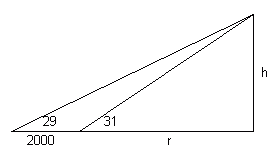A survey team is trying to estimate the height of a mountain above a level plain. From one point on the plain, they observe that the angle of elevation to the top of the mountain is 29 degrees. From a point 2000 feet closer to the mountain along the plain, they find that the angle of elevation is 31 degrees. How high (in feet) is the mountain? Hi Briana. You would use the tangent function to calculate this. Remember that you are travelling along a plain, so that means horizontal. You want a height, which means vertical. You have a right angle therefore. Here is a diagram.Let's call the height h and the remaining distance from our nearer point to the internal base of the mountain r. Then tan 31 = h/r. But as well, tan 29 = h/(r+2000). Now you have two equations with two variables, so solve this one of the usual ways (such as substitution of r). Hope this helps, Stephen La Rocque.